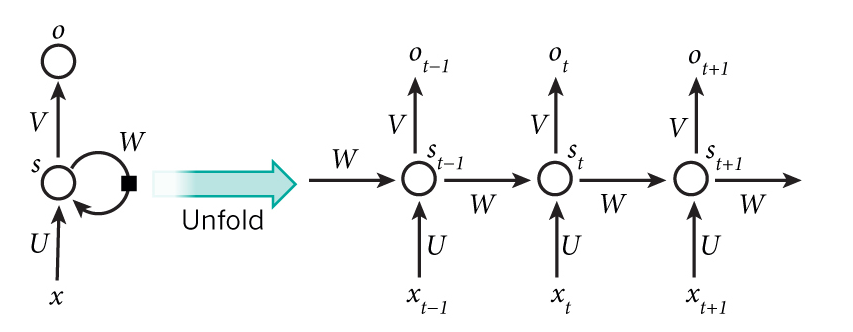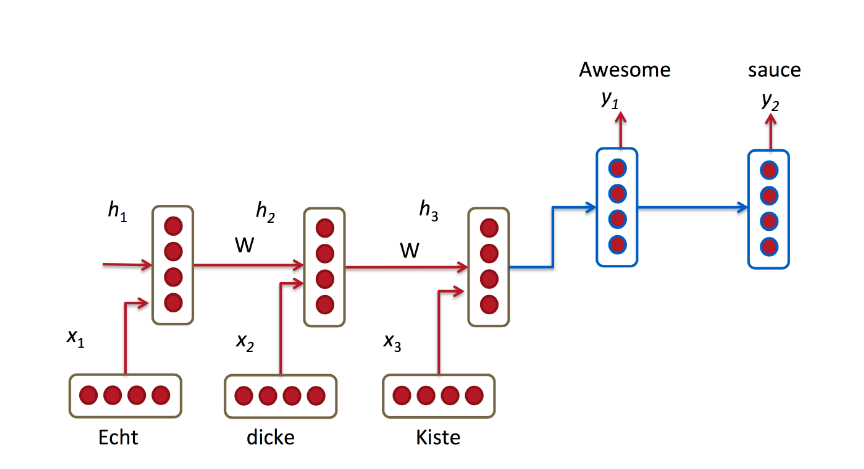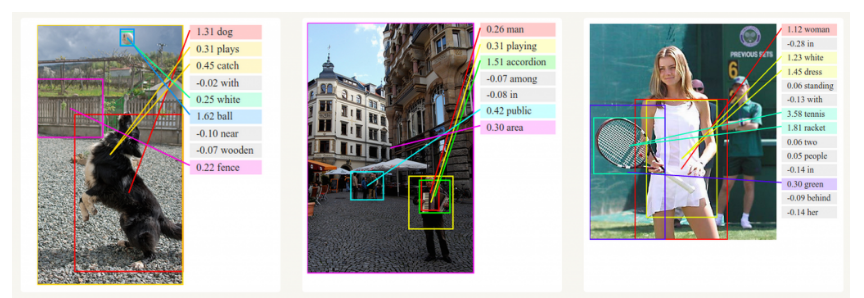RNN的思想是利用序列信息。在经典的神经网络中，我们认为所有的输入和输出是相互独立的。 但是对于许多其他任务这个想法是很不好的。如果你需要预测一个句子中的下一个字，知道前边的字会是很有帮助的。理论上RNN可以利用任意长度的序列的信息，但是实际只能处理很有限的前几步信息。下边是一个典型的RNN图示：$x_t$是第t步的输入，$x_1$可能是一个one-hot编码的的字。

$s_t$是第t步的隐藏状态，是网络的记忆功能。$s_t$是通过前边的隐藏状态和当前输入步计算得出：$st=f(Uxt+Ws_{t-1})$, f通常是tanh或者ReLU

$o_t$是第t步的输出，$ot=softmax(Vs_t)$

RNN的数学描述：

$s_t=tanh(Uxt+Ws_{t-1})$

$ot=softmax(Vs_t)$

$L(y,o)=-1/N\sum_{n}^{} y_nlogo_n$

RNN在很多NLP任务中表现的非常出色。

### 机器翻译根据原语言的输入序列，预测目标语言的序列达到翻译的目的。

### 生成文本描述和CNN一块，CNN识别图像内容，RNN生成图像的描述。